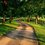# [Real Analysis] Does Uniform Convergence for a Sequence of Functions preserve Continuity?

If we have a sequence of functions $\{f_n\}$ that converges uniformly on $E\subset X$, and each $f_n$ is continuous on E, is the limit function

$f(x)=\lim_{n\to\infty}f_n(x)$ also continuous?

Let's fix $\epsilon>0$. Since we know that the sequence of functions $\{f_n\}$ is uniformly convergent on E, we can find an integer N so that $n\geq N$ implies

$\mid f_n(x) - f(x) \mid < \frac{\epsilon}{3}$

for all $x\in E$.

Additionally, we know that each of these functions $f_n$ is continuous, so for all $\epsilon>0$ we can find a $\delta>0$ so that

$\mid f_n(x) - f_n(p)\mid<\frac{\epsilon}{3}$

if $\mid x -p\mid < \delta$ for all $p$ in $E$.

Then the following inequality $\mid f(x)-f(p)\mid ~\leq ~\mid f(x) - f_n(x)\mid + \mid f_n(x)-f_n(p)\mid + \mid f_n(p) - f(p)\mid$

shows that for $n \geq N$, $\mid f(x)-f(p)\mid ~<~ \frac{\epsilon}{3}+\frac{\epsilon}{3}+\frac{\epsilon}{3 ~}=~ \epsilon$ whenever $\mid x - p \mid < \delta$.

Therefore, we conclude that the limit function $f(x)=\lim_{n\to\infty}f_n(x)$ is continuous on $E$ under the following conditions.Note by Bright Glow
3 years, 2 months ago

This discussion board is a place to discuss our Daily Challenges and the math and science related to those challenges. Explanations are more than just a solution — they should explain the steps and thinking strategies that you used to obtain the solution. Comments should further the discussion of math and science.

When posting on Brilliant:

• Use the emojis to react to an explanation, whether you're congratulating a job well done , or just really confused .
• Ask specific questions about the challenge or the steps in somebody's explanation. Well-posed questions can add a lot to the discussion, but posting "I don't understand!" doesn't help anyone.
• Try to contribute something new to the discussion, whether it is an extension, generalization or other idea related to the challenge.

MarkdownAppears as
*italics* or _italics_ italics
**bold** or __bold__ bold
- bulleted- list
• bulleted
• list
1. numbered2. list
1. numbered
2. list
Note: you must add a full line of space before and after lists for them to show up correctly
paragraph 1paragraph 2

paragraph 1

paragraph 2

[example link](https://brilliant.org)example link
> This is a quote
This is a quote
    # I indented these lines
# 4 spaces, and now they show
# up as a code block.

print "hello world"
# I indented these lines
# 4 spaces, and now they show
# up as a code block.

print "hello world"
MathAppears as
Remember to wrap math in $$ ... $$ or $ ... $ to ensure proper formatting.
2 \times 3 $2 \times 3$
2^{34} $2^{34}$
a_{i-1} $a_{i-1}$
\frac{2}{3} $\frac{2}{3}$
\sqrt{2} $\sqrt{2}$
\sum_{i=1}^3 $\sum_{i=1}^3$
\sin \theta $\sin \theta$
\boxed{123} $\boxed{123}$

Sort by:

What have you tried to do? For a fixed $x$ and $y$, how can we get from $f(x)$ to $f(y)$ with the least amount of error.

Staff - 3 years, 2 months ago

Thanks for your comment. Following your suggestion, I have shown that $f$ is continuous for a fixed $x$ and $p$ of $E$.

- 3 years, 2 months ago

The follow up question is: Is $f$ uniformly continuous?

Staff - 3 years, 2 months ago

That's a good question! I believe the limit function $f$ will only be uniformly continuous if the each $f_n$ is uniformly continuous.

- 3 years, 2 months ago

Yes indeed. A similar approach works.

Can you find a counterexample, namely a sequence of continuous $f_n$ that converge uniformly, but whose limit is not uniformly continuous?

Staff - 3 years, 2 months ago

The framework of what you proved is very unclear. Here’s what it is: Let $X,Y$ be bounded metric spaces, and assume that $Y$ is complete under its metrisation. Let $C_{u}(X,Y)$ be the space of uniformly continuous functions. It can be shown that this space is complete under the metric $d_{\infty}(f,g)=\sup\{d(f(x),g(x)):x\in X\}$. So, yes, it is well known that if $(f_{n})_{n}$ is Cauchy, then it converges to $\lim_{n}f_{n}$ (pointwise limit), and that this object itself is (uniformly!) continuous.

Here’s the proof. Let $(f_{i})_{i}\subseteq C_{u}(X,Y)$ be a $d_{\infty}$-Cauchy net. Then it is clear that the net is also pointwise convergent, since by definition $d(f_{i}(x),f_{j}(x))\leq d_{\infty}(f_{i},f_{j})\longrightarrow_{i,j}0$ for all $x\in X$. Since $Y$ is complete under its metrisation, $f(x):=\lim_{i}f_{i}(x)\in Y$ exists for all $x\in X$. We will now show that $f$ is uniformly continuous, and furthermore that $f_{i}\longrightarrow f$ within $C_{u}(X,Y)$.

Uniform continuity. Fix $\varepsilon>0$. Since the net is Cauchy, there exists a sufficiently large index $i_{0}$, such that ($\star$) $d_{\infty}(f_{i},f_{j})<\frac{\varepsilon}{4}$ for all $i,j\geq i_{0}$. Since $f_{i_{0}}\in C_{u}(X,Y)$ there exists $\delta>0$ such that ($\star\star$) $\forall{x,x'\in X:~}d(x,x')<\delta\Rightarrow d(f_{i_{0}}(x),f_{i_{0}}(x'))<\frac{\varepsilon}{4}$. Let $x,x'\in X$ with $d(x,x')<\delta$. By ($\star$) and ($\star\star$) one has $d(f_{i}(x),f_{i}(x'))<\frac{3\varepsilon}{4}$ for all $i\geq i_{0}$. Taking limits one has $d(f(x),f(x'))\leq\frac{3\varepsilon}{4}<\varepsilon$. Since $\varepsilon>0$ was arbitrarily chosen, this shows that $f$ is uniformly continuous.

Convergence. Let $\varepsilon>0$. Since the net is Cauchy, there exists a sufficiently large index $i_{0}$, such that $d_{\infty}(f_{i},f_{j})<\frac{\varepsilon}{2}$ for all $i,j\geq i_{0}$. For any $x\in X$ and $i\geq i_{0}$ it thus follows that $d(f(x),f_{i}(x))=\lim_{j}d(f_{j}(x),f_{i}(x))\leq\limsup_{j}d_{\infty}(f_{j},f_{i})\leq\frac{\varepsilon}{2}$. Hence for all $i\geq i_{0}$, taking supremums yields $d_{\infty}(f,f_{i})\leq\frac{\varepsilon}{2}<\varepsilon$. Since $\varepsilon>0$ was arbitrarily chosen, this shows that $f_{i}\longrightarrow f$ wrt the topology of uniform convergence.

- 3 years, 2 months ago